Repeated Confidence Intervals

In a group sequential test, repeated confidence intervals for a parameter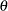are defined as a sequence of intervals,, for which a simultaneous coverage probability is maintained (Jennison and Turnbull 2000, p. 189). That is, asequence of repeated confidence intervals hasThese confidence limits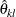andcan be created from observed statistic and boundary values at each stage.

### Two-Sided Repeated Confidence Intervals

Two sequences of repeated confidence intervals can be derived for a two-sided test. One is a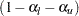rejection repeated confidence intervals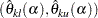,, and the other is aacceptance repeated confidence intervals,, where
andare the lower and upper Type I error probabilities for the test andand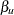are the lower and upper Type II error probabilities for the test (Jennison and Turnbull 2000, p. 196).

The rejection lower and upper repeated confidence limits at stageare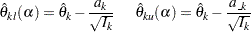The hypothesis is rejected for upper alternative if the lower limitand is rejected for lower alternative if the upper limit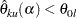. That is, the hypothesis is rejected if bothandare not in a rejection repeated confidence interval.

The acceptance lower and upper repeated confidence limits at stageare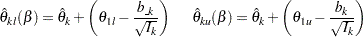The hypothesis is accepted if the lower limitand the upper limit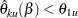. That is, a repeated confidence interval is contained in the interval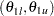.

### One-Sided Repeated Confidence Intervals

Like the two-sided repeated confidence intervals, two sequences of repeated confidence intervals can be derived for a one-sided test. Suppose the one-sided test has an upper alternative. Then one sequence of repeated confidence intervals is arejection repeated confidence intervals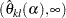,, and the other is aacceptance repeated confidence intervals,, whereandare the upper Type I and Type II error probabilities for the test. Thus, a sequence of repeated confidence intervals with confidence level greater than or equal tois given by.

The rejection lower repeated confidence limit and the acceptance upper repeated confidence limit at stageare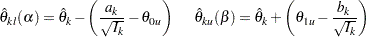The hypothesis is rejected if the lower limit. and it is accepted if the upper limit.